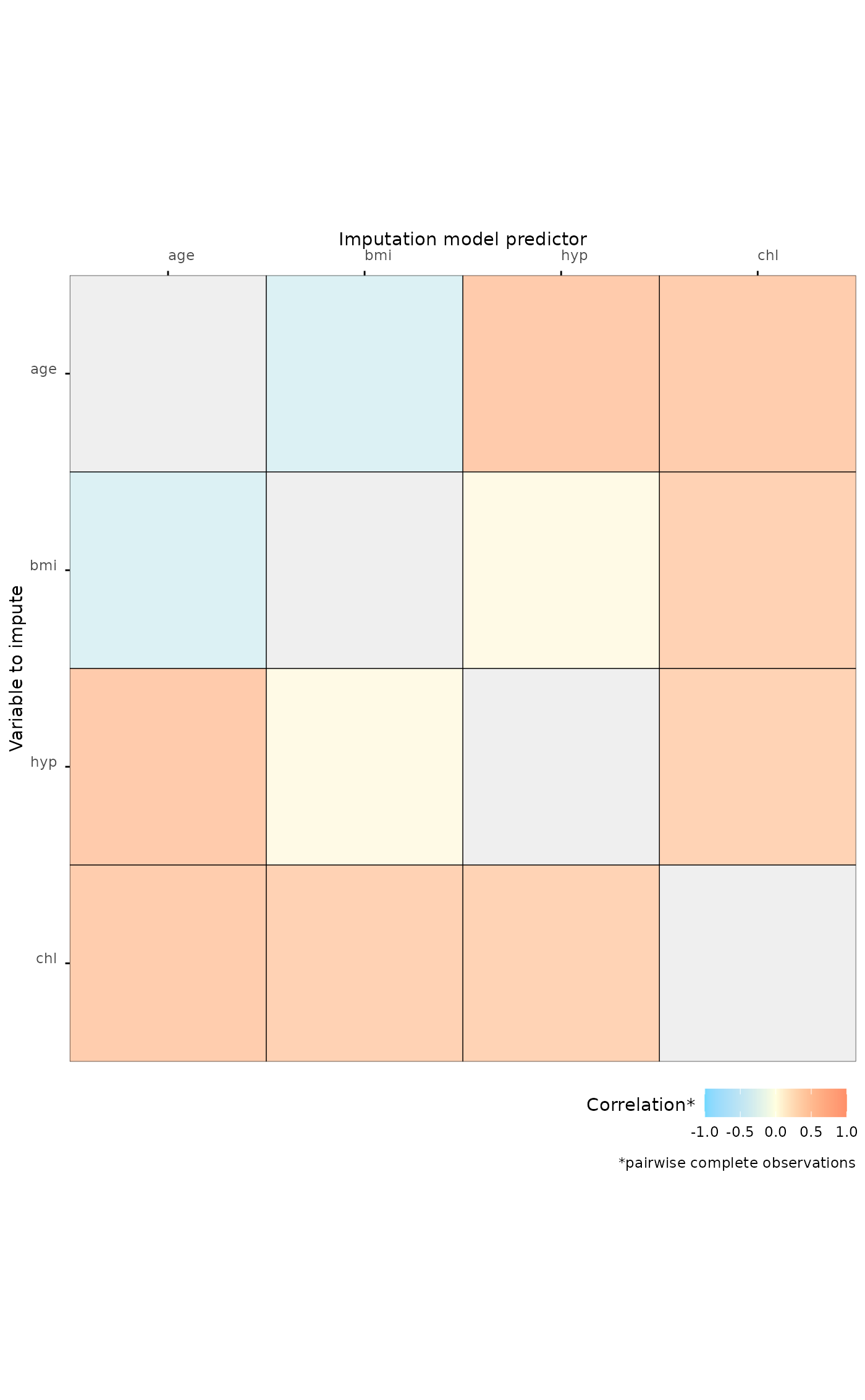Plot correlations between (incomplete) variables

## Usage

plot_corr(
data,
vrb = "all",
label = FALSE,
square = TRUE,
diagonal = FALSE,
rotate = FALSE,
caption = TRUE
)

## Arguments

data

A dataset of class data.frame, tibble, or matrix.

vrb

String, vector, or unquoted expression with variable name(s), default is "all".

label

Logical indicating whether correlation values should be displayed.

square

Logical indicating whether the plot tiles should be squares.

diagonal

Logical indicating whether the correlation of each variable with itself should be displayed.

rotate

Logical indicating whether the variable name labels should be rotated 90 degrees.

caption

Logical indicating whether the figure caption should be displayed.

## Value

An object of class ggplot2::ggplot.

## Examples

plot_corr(mice::nhanes, label = TRUE)